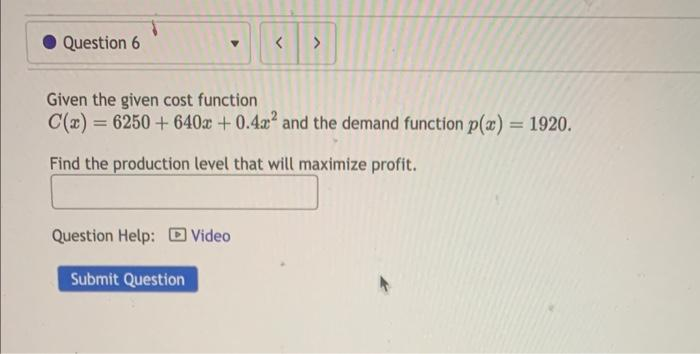Home / Expert Answers / Calculus / given-the-given-cost-function-c-x-6250-640x-0-4x2-and-the-demand-function-p-x-1920-find-the-prod-pa491

# (Solved): Given the given cost function C(x)=6250+640x+0.4x2 and the demand function p(x)=1920. Find the prod ...Given the given cost function and the demand function . Find the production level that will maximize profit. Question Help:

We have an Answer from Expert